SSC  >  Test: Surveying- 3

# Test: Surveying- 3

Test Description

## 20 Questions MCQ Test Mock Test Series of SSC JE Civil Engineering | Test: Surveying- 3

Test: Surveying- 3 for SSC 2023 is part of Mock Test Series of SSC JE Civil Engineering preparation. The Test: Surveying- 3 questions and answers have been prepared according to the SSC exam syllabus.The Test: Surveying- 3 MCQs are made for SSC 2023 Exam. Find important definitions, questions, notes, meanings, examples, exercises, MCQs and online tests for Test: Surveying- 3 below.
Solutions of Test: Surveying- 3 questions in English are available as part of our Mock Test Series of SSC JE Civil Engineering for SSC & Test: Surveying- 3 solutions in Hindi for Mock Test Series of SSC JE Civil Engineering course. Download more important topics, notes, lectures and mock test series for SSC Exam by signing up for free. Attempt Test: Surveying- 3 | 20 questions in 12 minutes | Mock test for SSC preparation | Free important questions MCQ to study Mock Test Series of SSC JE Civil Engineering for SSC Exam | Download free PDF with solutions
 1 Crore+ students have signed up on EduRev. Have you?
Test: Surveying- 3 - Question 1

### A scale of 1 inch = 50 ft. is mentioned on an old map. What is the corresponding equivalent scale?

Detailed Solution for Test: Surveying- 3 - Question 1

1 feet = 12 inches

1 inch = 2.54 cm

50 feet = 12 x 50 x 2.54 cm = 1524 cm = 15.24 m

So, 2.54 cm = 15.24 m

Hence 1 cm = 6 m

Test: Surveying- 3 - Question 2

### The needle of a magnetic compass is generally supported on a:

Test: Surveying- 3 - Question 3

### Identify the incorrect statement:

Detailed Solution for Test: Surveying- 3 - Question 3

Bench mark is a permanent reference point whose R.L is always known to us.

It is our first step to adjust the level for its temporary adjustment (centering, levelling) before start to take reading from a level.

Change point is a point which denotes shifting of level either due to inability to take reading or due to some obstruction.

Test: Surveying- 3 - Question 4

An object on the top of a hill 100 m high is just visible above the horizon from a station at sea level. The distance between the station and the object is:

Detailed Solution for Test: Surveying- 3 - Question 4

Distance between the station and the object is given by: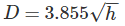Where,

h = height of visible horizon (meters)

D = Distance of visible horizon (km)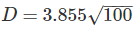D = 38.53 km.

Test: Surveying- 3 - Question 5

If every one of the three angles of a triangle have a probable error of ±1′′, then what will be the probable error in the sum of the measured internal angles of the triangle?

Detailed Solution for Test: Surveying- 3 - Question 5

Let probable error in angle “A” is = σA = ±1′′

probable error in angle “B” is = σB = ±1′′

Probable error in angle “C” is = σC = ±1′′

Probable error in the sum of the measured internal angles of the triangle is: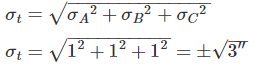Test: Surveying- 3 - Question 6

Direct ranging is possible only when the end stations are:

Detailed Solution for Test: Surveying- 3 - Question 6

The process of establishing or developing intermediate points between two terminal points or end points on a straight line is known as ranging.

Direct Ranging: The ranging in which intermediate ranging rods are placed in a straight line by direct observation from either end.

Direct ranging is possible only when the end stations are intervisible.

Test: Surveying- 3 - Question 7

Which type of errors introduces due to clogging of chain rings with mud?

Detailed Solution for Test: Surveying- 3 - Question 7

Due to clogging of chain rings with mud, actual length of the chain will become less than nominal length of chain. Therefore, measured value is greater than true value.

Error in measurement = Measured value – True value

Error = +ve

Test: Surveying- 3 - Question 8

If whole circle bearing of any line is W1, that of the preceding line is W2 and “d” is the deflection angles to the right, then choose the correct expression:

Test: Surveying- 3 - Question 9

The back sight reading on bench mark of R.L 500.00 m is 2.685 m. If foresight reading on the point is 1.345 m, the reduced level of the point is?

Test: Surveying- 3 - Question 10

The following consecutive readings were taken with a dumpy level and a 3 m staff on a continuous sloping ground:

0.425, 1.035, 1.950, 2.360, 2.950, 0.750, 1.565, 2.455.

Which of the following readings are back sights?

Test: Surveying- 3 - Question 11

A correction for error due to refraction is:

Test: Surveying- 3 - Question 12

If cross sectional area of an embankment at 30 m intervals are 20, 40, 60, 50 and 30 m2 respectively, then volume of the embankment on the basis of prismoidal rule is:

Detailed Solution for Test: Surveying- 3 - Question 12

Volume of embankment is given by: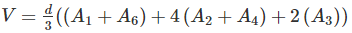d = 30 m

A1 = 20 m2

A2 = 40 m2

A3 = 60 m2

A= 50 m2

A= 30 m2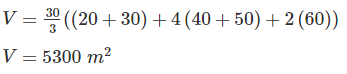Test: Surveying- 3 - Question 13

To orient a plane table at a point with two inaccessible points, the method generally adopted is:

Detailed Solution for Test: Surveying- 3 - Question 13

Two Point Problem: It is basically a resection method. In the two-point problem, two points are sighted from other point corresponding to the points given in plane table sheet.

In the method of intersection, the plotted position of stations is known, and the plotted position of objects are obtained by intersection.

In the method of resection, the plotted position of objects is known, and the plotted position of station is obtained.

Test: Surveying- 3 - Question 14

The indirect method of contouring has all the following advantages over direct method except:

Detailed Solution for Test: Surveying- 3 - Question 14

Direct Method v/s Indirect Method of contouring: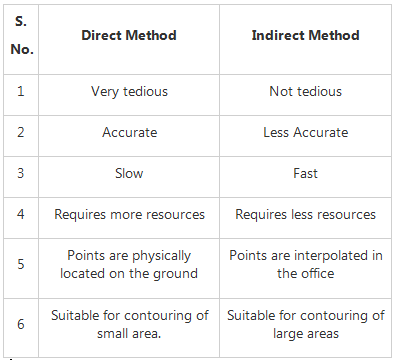Test: Surveying- 3 - Question 15

The values of whole circle bearing vary from ________.

Detailed Solution for Test: Surveying- 3 - Question 15

The values of the whole circle Bearing varies from 0° to 360° and is always taken with respect to North.

Test: Surveying- 3 - Question 16

If the degree of curve is 1, then radius of the curve for 30 m chain is

Test: Surveying- 3 - Question 17

A dumpy level is set up with its eyepiece vertically over a peg A. The height from the top of peg A to the centre of the eyepiece is 1.540 m and the reading on peg B is 0.705 m. The level is then set up over B. The height of the eyepiece above peg B is 1.490 m and a reading on A is 2.195 m. The difference in level between A and B is ________.

Test: Surveying- 3 - Question 18

A level when set up 25 m from peg A and 50 m from peg B reads 2.847 m on staff held on A and 3.462 m on staff held on B, keeping bubble at its centre while reading. If the reduced levels of A and B are 283.665 m and 284.295 m respectively, what is the collimation error per 100.0 m?

Detailed Solution for Test: Surveying- 3 - Question 18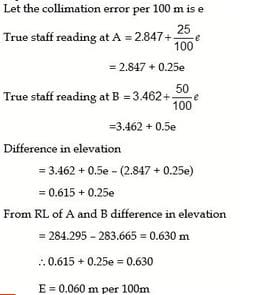Test: Surveying- 3 - Question 19

A 30 m metric chain is found to be 20 cm too long throughout a measurement. If the distance measured is recorded as 300 m, what is the actual distance?

Detailed Solution for Test: Surveying- 3 - Question 19

True length of chain × true measurement = incorrect length of chain × incorrect measurement

Hence, True measurement =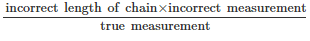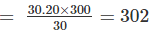Test: Surveying- 3 - Question 20

The difference between face left and face right observation of a theodolite is 3’. The error is:

Detailed Solution for Test: Surveying- 3 - Question 20

Error is half the difference between the face left and face right observation.

Error = 3'/2 = 1'30"

## Mock Test Series of SSC JE Civil Engineering

1 videos|1 docs|64 tests
Information about Test: Surveying- 3 Page
In this test you can find the Exam questions for Test: Surveying- 3 solved & explained in the simplest way possible. Besides giving Questions and answers for Test: Surveying- 3, EduRev gives you an ample number of Online tests for practice

## Mock Test Series of SSC JE Civil Engineering

1 videos|1 docs|64 tests(Scan QR code)